# IB DP Physics: HL复习笔记11.1.3 Faraday’s Law

• Faraday's Law connects the rate of change of flux linkage with induced e.m.f
• It is defined in words as:

The magnitude of the induced e.m.f is directly proportional to the rate of change of magnetic flux linkage

• Faraday's Law as an equation is defined as: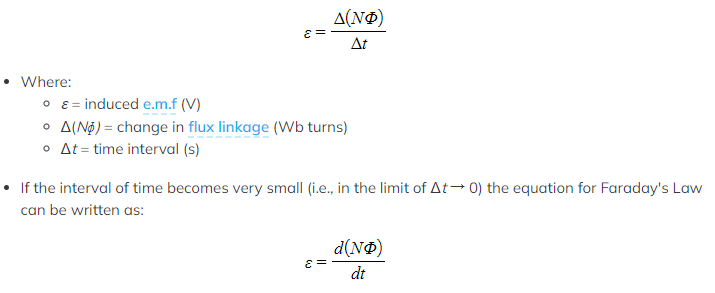Problems Involving Faraday’s Law
• Lenz’s law combined with Faraday’s law is given by the equation:
•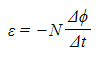This equation shows:
• When a bar magnet goes through a coil, an e.m.f is induced within the coil due to a change in magnetic flux
• A current is also induced which means the coil now has its own magnetic field
• The coil’s magnetic field acts in the opposite direction to the magnetic field of the bar magnet (shown by the minus sign)
• If a direct current (d.c) power supply is replaced with an alternating current  (a.c) supply, the e.m.f induced will also be alternating with the same frequency as the supply

#### Worked Example

A small rectangular coil contains 350 turns of wire. The longer sides are 3.5 cm and the shorter sides are 1.4 cm.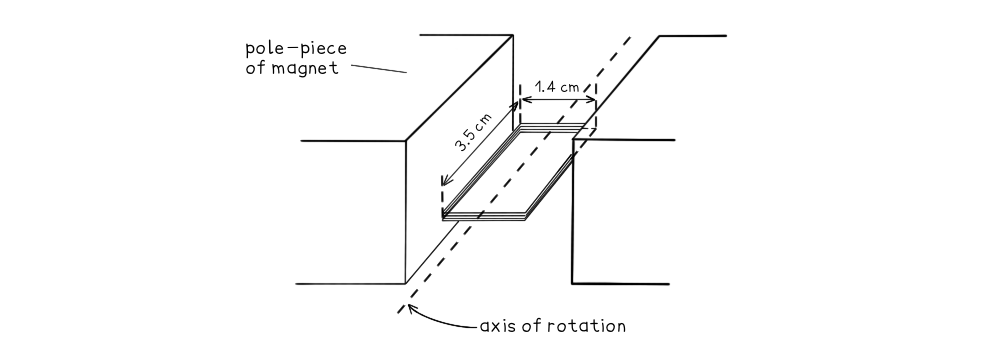The coil is held between the poles of a large magnet so that the coil can rotate about an axis through its centre. The magnet produces a uniform magnetic field of flux density 80 mT between its poles. The coil is positioned horizontally and then turned through an angle of 40° in a time of 0.18 s.

Calculate the magnitude of the average e.m.f induced in the coil.

Step 1: Write down the known quantities

• Magnetic flux density, B = 80 mT = 80 × 10-3 T
• Area, A = 3.5 × 1.4 = (3.5 × 10-2) × (1.4 × 10-2) = 4.9 × 10-4 m2
• Number of turns, N = 350
• Time interval, Δt = 0.18 s

Step 2: Write out the equation for Faraday’s law: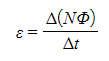Step 3: Write out the equation for the change in flux linkage:

• The number of turns N and the coil area A stay constant
• The flux through the coil changes as it rotates
• Therefore, the change in flux linkage can be written as:

Δ(NΦ) = NA(ΔB)

Step 4: Determine the change in magnetic flux linkage

• The initial flux through the coil is zero (flux lines are parallel to the coil face)
• The final flux through the coil is 80 mT (flux lines are perpendicular to the coil face)
• This is because the coil begins horizontally in the field and is rotated 90°
• Therefore, the change in flux linkage is:

Δ(NΦ) = NA(ΔB) = 350 × (4.9 × 10-4) × (80 × 10-3) = 0.014 Wb turns

Step 5: Substitute change in flux linkage and time into Faraday’s law equation: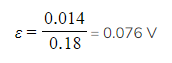Exam Tip

The 'magnitude' of the e.m.f just means its size, rather than direction. This is often what is required in exam questions, so the minus sign in Lenz's law is often not needed in calculations.

However, you may be expected to explain the significance of the minus sign. Be prepared to interpret it as an expression of Lenz's Law. You can find this is described on the next page.